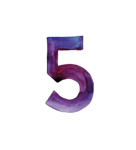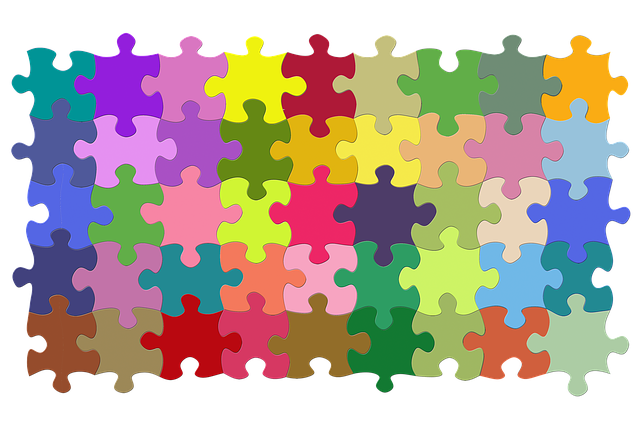# Logic Puzzles

Computational Thinker### Overview​

Computational thinking is a powerful skill that students need to learn. The benefits of being a good logical thinker and problem solver will help in school and in the workplace. To begin, students need to recognize patterns and sequences, create algorithms (step-by-step instructions or formulas) and devise logic for finding and fixing errors.

A great place to start is puzzles! Students love to solve puzzles and it helps develop reasoning skills useful for programming, computer science, and anything they might do. The teacher can start with very basic puzzles to those that students must solve for beginning coding.

Students will:

• Be able to solve simple to more difficult puzzles.
• Understand that learning to solve puzzles will help solve future problems.

Vocabulary Words:

1. Computational Thinking: Computational Thinking is the thought processes involved in formulating a problem and expressing its solution(s) in such a way that a computer-human or machine—can effectively carry out.
2. Algorithm: An algorithm is a detailed step-by-step instruction set or formula for solving a problem or completing a task

To prepare for this activity:

• The teacher will need to familiarize themselves with computational thinking. The teacher is not expected to teach all the aspects of computational thinking during this activity. Students need to learn logical thinking skills so they are prepared when they begin to code for the computer. Solving puzzles is the first step in the thinking process.

• Read the article from the BBC on computational thinking.

• Watch the video on Computational Thinking from Jules.

• In the classroom, the teacher may already have an assortment of puzzle books for the students to work on during free time. Make sure these are available for students.

• Here are some excellent puzzles to print out:
• If the students are already familiar with basic logic then have them solve some of the problems in the Computational Thinking puzzle book from CS4N. Download the puzzle book for free.

• A fun puzzle is the matchstick logic puzzle. You take nine matchsticks and create three triangles. Then you must create five triangles by only moving three matchsticks. Watch the video to learn how to solve the puzzle. For the classroom, the students could use popsicle sticks instead.

See the Accommodations Page and Charts on the 21things4students website in the Teacher Resources.

Directions for this activity:

1. The teacher will introduce the basic concepts of Computational thinking if the students are not familiar with it.
2. Show the video about Computational Thinking to the students.
3. Explain that the first step in learning basic coding skills is learning to apply logic to solve problems.
4. Print out several of the puzzles for the students to work on in class or as homework.

Optional Activities:

1. Have the students solve the Matchstick (popsicle) Logic Puzzle.
2. Solve several of the CS4N puzzles together as a class or as individuals.

Different options for assessing the students:

• Observations
• Check for understanding
• Students will solve the puzzles accurately.

MITECS: Michigan adopted the "ISTE Standards for Students" called MITECS (Michigan Integrated Technology Competencies for Students) in 2018.

Computational Thinker
5a. Students formulate problem definitions suited for technology-assisted methods such as data analysis, abstract models and algorithmic thinking in exploring and finding solutions.
5b. Students collect data or identify relevant data sets, use digital tools to analyze them, and represent data in various ways to facilitate problem-solving and decision-making.
5c. Students break problems into component parts, extract key information, and develop descriptive models to understand complex systems or facilitate problem-solving.
5d. Students understand how automation works and use algorithmic thinking to develop a sequence of steps to create and test automated solutions.

##### CONTENT AREA RESOURCES
• Story problems are great for reading comprehension.

• Word find puzzles will help with spelling and vocabulary.

• Crossword puzzles help with logic and vocabulary.

Students can solve math problem puzzles.

Credits
This task card was created by Melissa White, 21Things Project Manager, REMC Association of Michigan, October 2020. Updated October 2023.